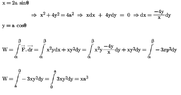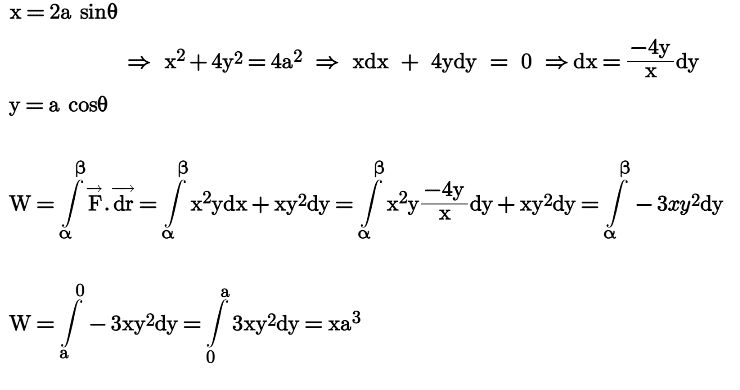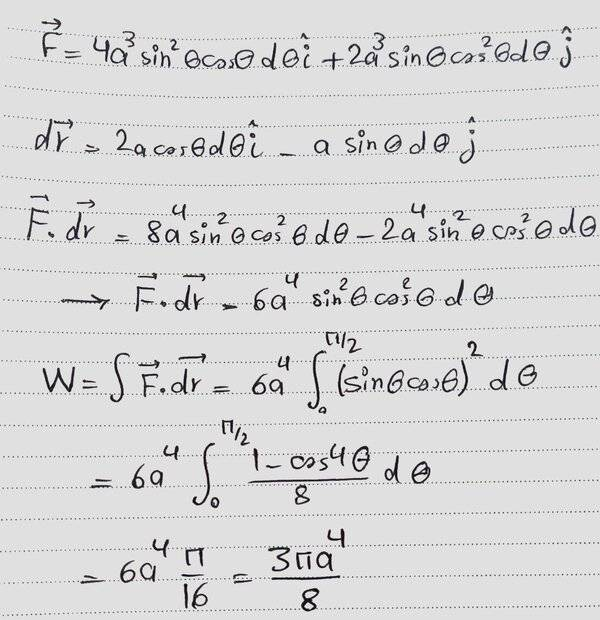# Work done by variable force

• MatinSAR
Yeah, you replace it with whatever variable you want.the equation of path is x=(2a) sinθ and y=(a) cosθWhat is ##\theta## here? It can't be the argument parameter of the polar representation of (x,y) since we would deduce ##\tan^2(\theta)=\frac 12##. So I assume it is an arbitrary parameter unrelated to polar coordinates. But then, where do the integration bounds come from?I see no diagram.f
LaTeX is preferable, but if your work is too involved, you can post a picture as long as it is clearly legible and right side up. Use the "Attach files" link, lower left.
Thank you ... I have done it with a math editor.

#### Attachments

•1121212.png
8.8 KB · Views: 37
I don't get the factor as 6. Did you handle the signs correctly? The rest looks fine.
What about Theta ?! Did I specify theta correctly?
I get the factor 6 again ! Let me send a picture ...

I'm getting what you are getting.

$$\int 3 x y^2 dy = \int 3 \cdot y^2 \cdot 2 \sqrt{a^2 - y^2} dy$$

Let:

##\frac{y}{a} = \sin \beta##
## \frac{ \sqrt{a^2 - y^2} }{a} = \cos \beta##

It follows that

$$y^2 = a^2 \sin^2 \beta$$
$$\sqrt{a^2 - y^2} = a \cos \beta$$
$$dy = a \cos \beta d \beta$$

$$\implies \int 3 x y^2 dy = 6 a^4 \int \sin^2 \beta \cos^2 \beta d \beta$$

Last edited:
•MatinSAR
I don't get the factor as 6. Did you handle the signs correctly? The rest looks fine.
I also get a factor of 6, although I would never have done the math the way that he did it>

•MatinSAR
I also get a factor of 6, although I would never have done the math the way that he did it>
Was it true ?!
What was wrong with the way I have done the mathI'm getting what you are getting.

Was it true ?!
What was wrong with the way I have done the mathI didn't do the integral!

•MatinSAR
Post your work, it will get checked by someone...

•MatinSAR
What about Theta ?! Did I specify theta correctly?
I get the factor 6 again ! Let me send a picture ...
Yes, my mistake… I should have waited until I had the time to check properly.

•MatinSAR
Post your work, it will get checked by someone...It is a line integral, not a double integral, so you must arrange that there is only one variable in the integrand. If you want to do it as an integral wrt y then you must first replace all the occurrences of x with what x is as a function of y.
But really, the parametric approach using theta is much easier.

•MatinSAR and erobz
Show the work. Explain how you get the last result?

•MatinSAR
It is a line integral, not a double integral, so you must arrange that there is only one variable in the integrand. If you want to do it as an integral wrt y then you must first replace all the occurrences of x with what x is as a function of y.
But really, the parametric approach using theta is much easier.
So my answer is wrong , isn't it ?!
Thank you ... I will try to do it using parametric approach and I will send a picture of the work ...
Show the work. Explain how you get the last result?
Thank you ... I will try to do it using parametrich approach and I will send a picture of the work ...

•erobz
I will try to do it using parametric approach
I'm confused. Isn’t that what you already did in post #32? Except that you did not perform the integral.

•MatinSAR
The integration is very simple. It just involves the use of trigonometric identities.$$(\sin{\theta}\cos{\theta})^2=\frac{\sin^2{2\theta}}{4}=\frac{1-\cos{4\theta}}{8}$$

Last edited:
•MatinSAR
Hi @MatinSAR. Can I point out a fundamental mistake you are making? I don’t think anyone has mentioned it yet (apologies if they already have).

The final expression for W can not contain x or y (or θ). These are variables which change as you move along the path. They must disappear when the definite integral is evaluated.

You made the same mistake in your Post #1 solution, where your final expression for W contained x and y.

The expression for W can contains only given constants (here only the 'a') and pure numbers like 5, π and √2 (just picking random values as examples).

For example, if you determine the formula for the circumference of a circle, x²+y²=a², it wouldn’t make sense to have x and/or y in the final formula.

•MatinSAR
I'm confused. Isn’t that what you already did in post #32? Except that you did not perform the integral.
Yes. It was unfinished.
The integration is very simple. It just involves the use of trigonometric identities.$$(\sin{\theta}\cos{\theta})^2=\frac{\sin^2{2\theta}}{4}=\frac{1-\cos{4\theta}}{8}$$
Thank you.
Hi @MatinSAR. Can I point out a fundamental mistake you are making? I don’t think anyone has mentioned it yet (apologies if they already have).

The final expression for W can not contain x or y (or θ). These are variables which change as you move along the path. They must disappear when the definite integral is evaluated.

You made the same mistake in your Post #1 solution, where your final expression for W contained x and y.

The expression for W can contains only given constants (here only the 'a') and pure numbers like 5, π and √2 (just picking random values as examples).

For example, if you determine the formula for the circumference of a circle, x²+y²=a², it wouldn’t make sense to have x and/or y in the final formula.

•Steve4Physics
I hope it's finally true ...The force is conserative so If I choose another path the final answer shouldn't change, Is it true ?!

The force is conserative
Is it? What do you get if you integrate from 0 to 2π?

•MatinSAR
Is it?
It was mentioned in question.
Thank you.

.
.
.
.
.
.
I hope I haven't forgotten anyone.

Thank you for your help and time.•erobz
It was mentioned in question.
Try the path along the axes. Isn't F always zero there?

•MatinSAR
No it's not.
Oh yes it is! (If you familiar with British pantomime.)

Can I expand on what @haruspex said in Post #57?

Referring to the Post #21 diagram, suppose you take the following route:
- from A(0,a), move along the y-axis to the origin (0,0);
- from the origin (0,0) move along the x-axis to C(2a,0).

##\vec F = x^2y~\hat i + xy^2 \hat~j##

While moving along the y-axis (x=0); both components of ##\vec F## are zero.
Similarly while moving along the x-axis.

The total work done along this path is therefore zero. This is different to your calculated value for the original route from A to C.

So you can see (without doing any maths) that the force is not conservative.

•MatinSAR
Referring to the Post #21 diagram, suppose you take the following route:
- from A(0,a), move along the y-axis to the origin (0,0);
- from the origin (0,0) move along the x-axis to C(2a,0).
Great !
So it's not conserative ...
Thank you.